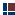See 1260d.com for most recent articles

The Temple and 1260 days of Bible Prophecy

Measuring the Tabernacle Curtains

(Chart of measurements)

(Based on Exodus 26)

Two "curtains" and two "coverings" covered the tabernacle, (Exodus 26). The total (linen) material used for the first curtain was 1120 (4 x 28 x 10) square cubits.

Curtains as 1320 square cubits:

In addition to the 1120 square cubits of linen material for the first curtain covering, there was an additional 100, plus 100 square cubits of this same fine linen used for the two entry veils that led into the Holy, and the Holy of Holies. (1120 + 100 + 100 = 1320 square cubits total.)

 See note about the implied, or at least idealized, size of the 2 veils, as based upon the fact that the front and back of the tabernacle was 10 by 10 cubits. No other definite measurements are given concerning the entrance, so the 10 by 10 area of the curtain is assumed. But the fact that the total amount of linen thereby matches the total amount of material for the next curtain (goat skins), strongly supports this assumption as  likely literally correct; but if not, then certainly idealistically speaking, remembering that the tabernacle is saturated with symbolism.

Thus, there was 1320 square cubits of fine linen used in the Tabernacle altogether. This is important because, '1320' is the same total as the next layer of "curtain" over the Tabernacle, this time made of goat’s hair; (i.e., 4 x 30 x 11 = 1320 square cubits also)! Moreover, this establishes a pattern for the remaining two coverings, which we can assume likewise had the same measurements since they had basically the same purpose, and no other measurements are given. Only the materials used, (and thus the symbolism), for the remaining two coverings are different; namely, "ram skins dyed red," and "sea cows".

Curtains as 1260 square cubits:

We just noted that the second (and remaining) curtains had a total area of 1320 square cubits. However, we are told that 60 square cubits of that was folded back. Everything in the Tabernacle is symbolic of something, (see the book of Hebrews). Aside from any practical purpose, why this peculiar folding back of 60 square cubits? This second curtain over the Tabernacle (made of goats hair) is said to have the last of its 11-sectioned curtain folded back. Since each of these 11 sections were 30 by 4 cubits ( = 120 square cubits), the total therefore is:

 1320 less 60 (that is, half of 120) = 1260 square cubits  (4 x 30 x 10.5 = 1260)

(Technical note: I strongly suspect that this half-fold backwards was actual sewn backwards, otherwise it would flop forward since it overhung the entrance to the Tabernacle by 4 (less 2) cubits. The purpose of the overhang appears to be for the purpose of creating an extra thick fold hanging down at the entrance so as to further obscure the interior to possible onlookers when a priest entered.)

Since the remaining two coverings were to overlap the first couplet of tent curtains, it follows that they would have used the same dimensions of 1260 square cubits, (i.e., 1320 less 60).

Thus we have two sets of coverings. The first two are called "curtains." The second two are simply called "coverings." Because 200 square cubits of the linen material was used for the entrance (veil) to the tabernacle rather than to cover it, and because the last 3 curtains/coverings were folded back 60 square cubits, the following is how we can calculate the four materials (each in 2 sets) used for the tabernacle. (Note: the first curtain was not folded back.)

Tabernacle measurements in original cubic cubits

(Exodus 26)

(This chart is repeated after this,
except with the meaning of each number included)

 Materials used 1st curtain fine linen 2nd curtain goats hair 1st covering red ram-skins 2nd covering sea cows Total ---including folds--- Total of all 4 materials 1320 (curtain and veils) 1320 1320 1320 5280 Subtotal 2640 Subtotal 2640 Curtains/coverings only 1120 1320 1320 1320 5080 Subtotal 2440 Subtotal 2640 ---not including folds--- Total of all 4 materials 1320 (curtain and veils) 1260 1260 1260 5100 Subtotal 2580 Subtotal 2520 Curtains/coverings only 1120 1260 1260 1260 4900 Subtotal 2380 Subtotal 2520 Inside wooden paneling 720 6000 Total of all 5 materials 1320 + 1320 + 1320 + 1320 + 720 = 6000Tabernacle: Temple of TimeMeasuring the Tabernacle CurtainsTabernacle Curtains as 1320 and 1260 sq. cubits
(The tabernacle and the 1260 days of bible prophecy. Also, 1290 and 1335 days and years.)Comparing Measurements of Tabernacle Curtains with Bible-Prophecy time spansMeasuring the Tabernacle Curtains: Total material usedMeasuring the Tabernacle Curtains: Detailed breakdownBible references that link the temple and tabernacle with timeOther Links Between Time and the Temple / TabernacleMeasuring Ark of the CovenantOverview of Other Bible TemplesTabernacle: Is the curtain veil 100 sq. cubits

Also see, How Israel became a nation in 1948 on the exact date predicted in the Bible,
1260 x 2 years from Ezekiel's temple!